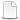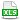HomeTechniques and Tips@RISK 6.x/7.x with ProjectsScheduling Rework in a Project

# 11.18. Scheduling Rework in a Project

Applies to: @RISK 6.x/7.x, Professional and Industrial Editions

When a certain task, call it Task 3, is finished, there's a 20% probability that the work may not be acceptable, in which case Task 3 will have to be redone. (The rework might include one or more predecessors of Task 3 too.) How do I set this up with probabilistic branching in @RISK?

Probabilistic branching can go only forward, not backward. You could have a rework task, as a successor to Task 3, and branch around that rework task with probability 100%–20% = 80%. However, there's a more straightforward solution: use a RiskProjectAddDelay function in your Risk Register.

In the attached example Rework, Tasks 2 and 3 get reworked (or not) as a group, with probability 20%. If the RiskBernoulli in the Risk register returns 1 in a particular iteration then for that iteration the RiskProjectAddDelay adds a task sandwiched in between Tasks 3 and 4. The duration of that rework task is the sum of the durations of Tasks 2 and 3, which is 19 days. Without reworking, the overall duration of the project is 21 days; with reworking Tasks 2 and 3 it's 21+19 = 40 days. If you run a simulation and look at the total duration in C2 on the Tasks sheet, you'll see that 80% of the time it's 21 days, and the other 20% it's 40 days.

In the example, almost everything is deterministic, just to make it easier to see how it all works. (A real project would most likely have probability distributions for the durations of Tasks 2, 3, and 4.) But you could get more elaborate. For example, maybe under some circumstances a second rework would be needed. In that case, replace the RiskBernoulli in C2 of the Risk Register with a RiskBinomial or other suitable discrete distribution.

Or maybe a rework doesn't take the same time as the original, but it could be more or less. For that, replace the duration in A2 of the Risk Register with a continuous distribution, perhaps something like

`=RiskVary(Tasks!C3+Tasks!C4,-10,15,0,,"Triang")`

as shown in the example Rework2. This is a triangular distribution varying between 15% below and 10% above the 19 days for Tasks 2 and 3 combined. Run a simulation. You'll see that the project duration is still 80% likely to be 21 days, but instead of a single value at 40 days there's now a distribution of possible durations around 40 days.

Last edited: 2015-09-01

•Rework.mpp
•Rework.xlsx
•Rework2.mpp
•Rework2.xlsx